# Network Reverse Engineering with Approximate Bayesian Computation

## Abstract

Elucidating regulatory network is an essential step towards understanding the normal cell physiology and complex pathological phenotype. Reverse-engineering consists of using measurements over time or over different experimental conditions to discover the structure of a network, for instance, in a targeted cellular process. In addition, many real data, such as gene expressions or protein abundances data, are usually noisy, highly correlated, and have high dimensionality, which explains the need for specific statistical methods to reverse engineer the underlying network. Among known methods, Approximate Bayesian Computation (ABC) algorithms have not been very well studied. Due to the computational overhead, their application is also limited to a small number of nodes. In this work, we have developed a new multilevel ABC approach that has less computational cost. At the first level, the method captures the global properties of the network, such as scale-freeness and clustering coefficients, whereas the second level is targeted to capture local properties, including the probability of each couple of nodes being linked.

### Keywords

Network Inference, Approximate Bayesian Computation, Bioinformatics

Classification/MSC: 62E17, 62F15, 62J07, 62P10, 92C42

## Introduction

The goal of networkABC is to provide an inference tool based on approximate Bayesian computation to decipher network data and assess the strength of their inferred links.

It is well known that such algorithms have been scarcely used in that setting and, due to the computational overhead, their application was limited to a small number of nodes.

On the contrary, our algorithm was made to cope with that issue and has a low computational cost. It is a new multi-level approximate Bayesian computation (ABC) approach.

It can be used, for instance, for elucidating regulatory network, which is an essential step towards understanding the normal cell physiology and complex pathological phenotype. Reverse-engineering consists of using measurements over time or over different experimental conditions to discover the structure of a network, for instance, in a targeted cellular process. The fact that many real data are usually noisy, highly correlated, and have high dimensionality explains the need for specific statistical methods to reverse engineer the underlying network.

## Findings

### Overview

Here is an overview of our main finding, the network ABC algorithm.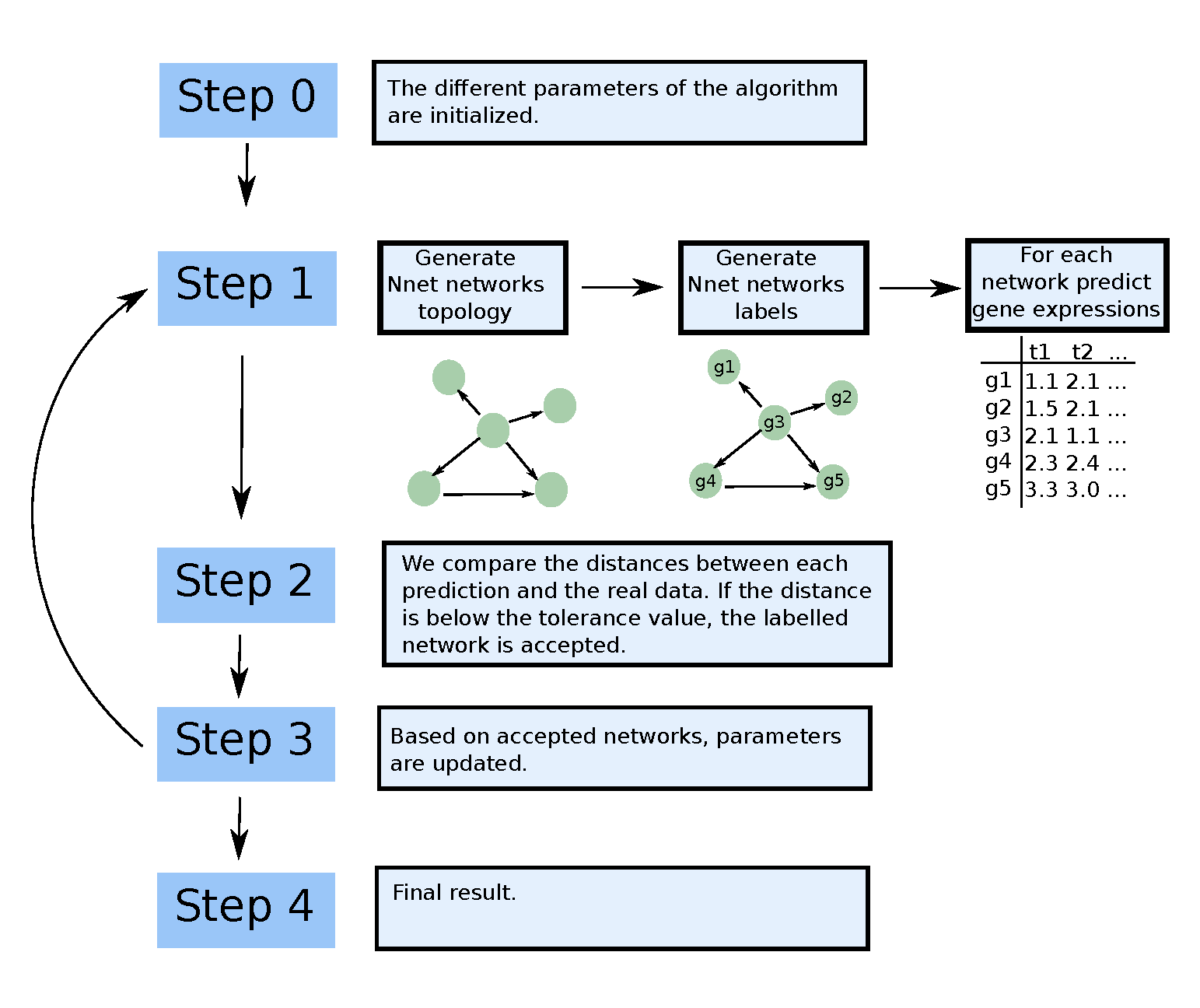### Step 0

Several parameters need to be specified; some are optional or may be determined using a preliminary run of the algorithm.

• At least a clustering coefficient value clust_coeffs. Defaults to c(0.33, 0.66, 1). More than one clustering coefficient may be specified.
• The tolerance tolerance is the maximal distance between simulated and real data to accept the network. Defaults to NA. If tolerance is not specified, then a first pass of the algorithm with a single iteration is made to find a relevant tolerance value.
• The number of hubs number_hubs. Defaults to 3.
• The number of iterations iterations. Defaults to 10.
• The number of networks number_networks simulated at each iteration. Defaults to 1000000
• Specify the a priori probability hub_probs for each node to be a hub. Defaults to NA, which means uniform probability.
• Specify the a priori probability neighbour_probs for each couple of nodes to be linked. Defaults to NA, which means uniform probability.
• is_probs needs to be set either to one if you specify hub_probs and neighbour_probs or to zero if neither probabilities are specified. Warning: both hub_probs and neighbour_probs should be specified if is_probs is one. If is_prob is zero, these arrays should indicate an array of a specified size.

### Step 1

The number of nodes and the targeted clustering coefficient should be specified in order to generate a network. In graph theory, a clustering coefficient measures the degree to which nodes in a graph tend to cluster together. More details on the global clustering coefficient can be found on the Wikipedia entry https://en.wikipedia.org/wiki/Clustering_coefficient

#### First level: Generation of a network topology

At the first level, the method captures the global properties of the network, such as scale-freeness and clustering coefficients, by generating a network topology that is partially based on the algorithm by Di Camillo, Barbara, Gianna Toffolo, and Claudio Cobelli. ”A gene network simulator to assess reverse engineering algorithms.” Annals of the New York Academy of Sciences.

#### Examples and checks

Load the networkABC package.

library(networkABC)


The following produces a network with 100 nodes and a targeted clustering coefficient of 0.33 :

net<-network_gen(100,0.33)


Then, we can plot the network :

require(network)

## Loading required package: network

## network: Classes for Relational Data
## Version 1.16.1 created on 2020-10-06.
## copyright (c) 2005, Carter T. Butts, University of California-Irvine
##                     Mark S. Handcock, University of California -- Los Angeles
##                     David R. Hunter, Penn State University
##                     Martina Morris, University of Washington
##                     Skye Bender-deMoll, University of Washington
##  For citation information, type citation("network").
##  Type help("network-package") to get started.

plot(network(net$network))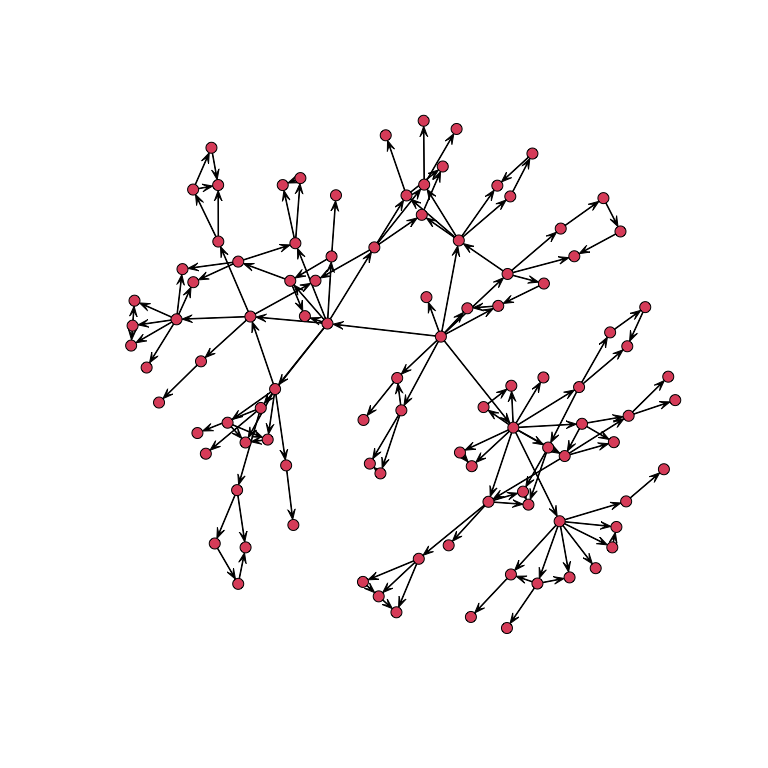Here is a simulation to show that the algorithm produces networks with a clustering coefficient close to the targeted one f<-function(a){ a<-a[!is.nan(a)] }  We generate 500 networks with 500 nodes with a targeted clustering coefficient of 0.33 set.seed(1234) clco<-rep(0,500) for(i in 1:500){ N<-network_gen(500,.33)$net
N<-N+t(N)
clco[i]<-mean(f(abs(networkABC::clusteringCoefficient(N))))
}


Here is the result :

mean(clco)

##  0.3306789

sd(clco)

##  0.02874285

ggplot2::qplot(clco)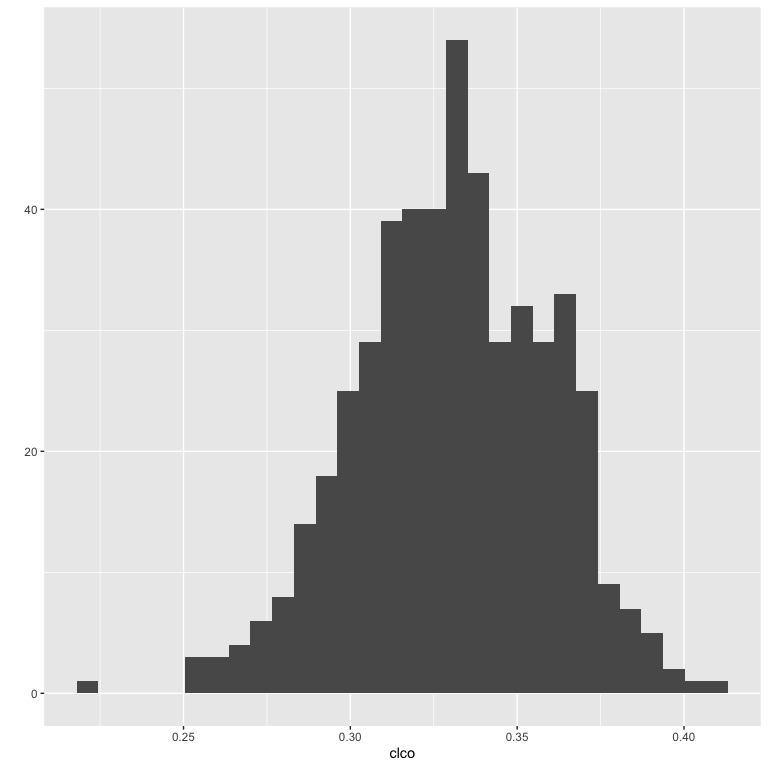Notice that the algorithm cannot reach all desired values of clustering coefficients because the network has to be scale-free.

For example, a clustering coefficient of 1 implies that every couple of nodes are linked together; such a network is not scale-free.

#### Second level: Capturing local propoerties

The second level is targeted to capture local properties, including the probability of each couple of nodes being linked.

Nodes are linked to each other through an iterative procedure, consisting of three main steps, explained in detail below.

Let us call $V$ the set of nodes to be connected in the graph $G$ at the current iteration $t$ and $H$ the set of nodes to be connected at iteration $t + 1$. $V$ is initialized as $V = {1, …,N}$, that is, with all the $N$ nodes in $G$, whereas $H$ is initialized as the empty set $H$.

1. Three candidate modules are generated. The structure is sampled from a pool of motifs, with the possibility of random changes. The number of nodes of the module is set at random. In this algorithm, we have feedback motif, feedforward motifs and loops.
2. A score is assigned to each module, and one of the three modules is sampled with probability proportional to this score; let us denote the sampled module by M and the number of its nodes by $m$.
3. $m$ nodes are sampled from $V$ and linked to each other in the graph $G$, according to the selected module structure $M$; $V$ is updated by deleting the $m$ sampled nodes; $H$ is updated by adding the nodes. At the end of this process, $V$ is empty, whereas $H$ is composed of many motifs. To link the motifs together, we have to choose one node in each motif that will be the first position. This set of nodes is then considered as set $V$.

$V$ is updated by deleting the $m$ sampled nodes; $H$ is updated by adding the nodes.

#### Data simulation

Data are simulated using the labelled network that was generated.

### Step 2

If the distance between the real data and the ones simulated using the labelled network is lower than the tolerance threshold, then the labelled network is accepted. Hence, a network is assessed as good if the data simulated using that network are close enough to the real observed data.

Go back to Step 1 until trying out number_networks networks.

### Step 3

Based on accepted networks, parameters are updated. If the number of done iterations is lower than iteration, increase the number of done iterations by one and go back to Step 1.

### Step 4

Return the results.

## Running the ABC algorithm

### The simpliest way

set.seed(123)
M<-matrix(rnorm(30),10,3)
result<-abc(data=M)

## First run of abc to find tolerance
## ===============================
## Iteration=1
## Accepted:1000
## Probabilities of clustering coefficients:
## 0.325000 0.349000 0.326000
## Tolerance value
##       5%
## 4.523488
## ===============================
## Beginning main run of abc
## ===============================
## Iteration=1
## Accepted:45
## Probabilities of clustering coefficients:
## 0.488889 0.311111 0.200000
## ===============================
## Iteration=2
## Accepted:109
## Probabilities of clustering coefficients:
## 0.522936 0.266055 0.211009
## ===============================
## Iteration=3
## Accepted:139
## Probabilities of clustering coefficients:
## 0.654676 0.201439 0.143885
## ===============================
## Iteration=4
## Accepted:163
## Probabilities of clustering coefficients:
## 0.705521 0.196319 0.098160
## ===============================
## Iteration=5
## Accepted:154
## Probabilities of clustering coefficients:
## 0.753247 0.181818 0.064935
## ===============================
## Iteration=6
## Accepted:210
## Probabilities of clustering coefficients:
## 0.819048 0.142857 0.038095
## ===============================
## Iteration=7
## Accepted:200
## Probabilities of clustering coefficients:
## 0.860000 0.120000 0.020000
## ===============================
## Iteration=8
## Accepted:163
## Probabilities of clustering coefficients:
## 0.865031 0.110429 0.024540
## ===============================
## Iteration=9
## Accepted:232
## Probabilities of clustering coefficients:
## 0.909483 0.077586 0.012931
## ===============================
## Iteration=10
## Accepted:238
## Probabilities of clustering coefficients:
## 0.907563 0.079832 0.012605


We can plot the results in three different ways :

networkABC::showHp(result)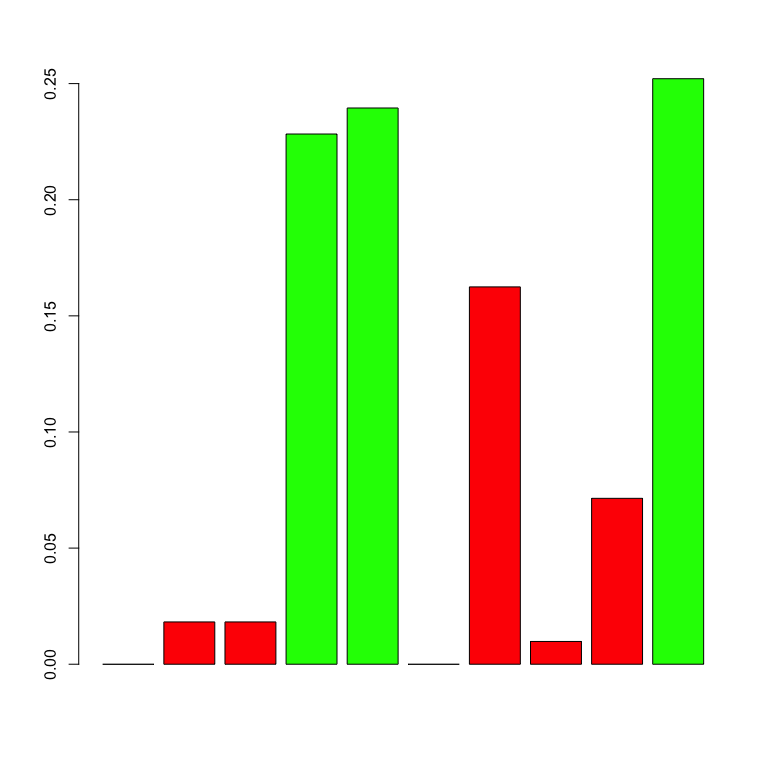##   gene.hubs hubs.proba
## 1         4  0.2282913
## 2         5  0.2394958
## 3        10  0.2521008


This plot show the probabilities for each node of being a hub. The following shows the probability for each couple of nodes of being linked :

showNp(result)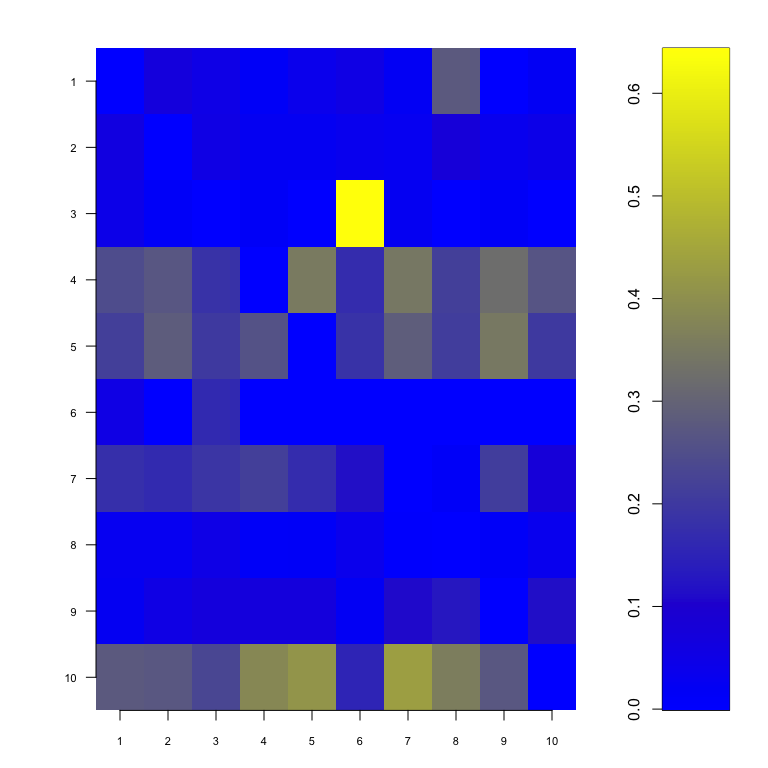Specifying a probability cutoff (the minimal probability for which we can say that two nodes are linked), we can plot the network :

showNetwork(result,0.3)In this plot, the diameter of a node increases with the number of its children whereas the color is a function of the probability for each node of being a hub.

You can also have a look on the error :

hist(result\$dist)### Using ABC algorithm with full options

You can specify all the arguments of the ABC function.

For example :

result<-abc(data=M,
clust_coeffs=0.33, #you can specify more than one clustering coefficient
tolerance=3.5, #maximal distance between simulated and real data
# to accept the network
number_hubs=3,#the number of hubs
iterations=10, #number of iterations
number_networks=1000000,#number of network simulated at each iteration
hub_probs=NA,#specify the a priori probabilty for each node to be a hub
neighbour_probs=NA,#specify the a priori probability for each couple
is_probs=1)#set this last option to one.


### Availability of supporting source code and requirements

List the following:

### Data availability

The dataset netsimul is the result of the network simulation function network_gen that is provided with the package.

The dataset resabc is the of an ABC inference that is provided with the package. More precisely, it is the for the reverse engineering of a simulated Cascade network.

## Declarations

### List of abbreviations

ABC: Approximate Bayesian Computation

None.

### Funding

We are grateful to Université de Strasbourg and CNRS for their former support.

### Author contributions

Frédéric Bertrand and Myriam Maumy designed and supervised the project then created the technical note.

### Acknowledgments

We are grateful to Nicolas Jung and Khadija Musayeva for their past contributions to the project.